ISSN ONLINE(2320-9801) PRINT (2320-9798)

All submissions of the EM system will be redirected to Online Manuscript Submission System. Authors are requested to submit articles directly to Online Manuscript Submission System of respective journal.

# Survey of One Time Signature Schemes on Cloud Computing

 Revatthy Krishnamurthy1, K.P. Kaliyamurthie2 M.Tech Scholar, Department of Computer Science & Engineering, Bharath University, Chennai, India Professor, Department of Computer Science & Engineering, Bharath University, Chennai, India. Related article at Pubmed, Scholar Google

Visit for more related articles at International Journal of Innovative Research in Computer and Communication Engineering

## Abstract

Cloud computing is a technology and large - scale computing resources to effectively integrate, and the resources are computed based on cryptographic secure hash functions. The biggest problem of one time signature scheme is the key management. An efficient key management is needed to make one-time signature scheme and the Merkle signature schemes feasible. This paper presents detailed study on one-time signature and Merkle signature schemes.

### KEYWORDS

Cloud computing, one-time signature scheme, Merkle signature scheme, Key generation.

### I. INTRODUCTION

Cloud is a large group of interconnected computers, which is a major change in how we store information and run application. Cloud computing is used for many bigdata applications and it is cost effective. Data storage and sharing services in the cloud with three entities such as the cloud, the third party Auditor (TPA), and users who participate as a group includes one original user and a number of group users.
The original user is an original owner of data, and shares data in the cloud with other users . A single message using a given piece of private or public information. The conventional signature schemes like RSA, the same key pair can be used to authenticate large number of documents. One-time signatures by Merklecalled Merklesignature scheme, which does not require new key pairs for each message. Simple digital signature scheme for fixed - length messages using a one-way function is Lamport's one-time signature scheme. Diffie OTS , the merkle OTS , the Winternitz OTS [4,5], etc are different signature schemes. The security of one-time signatures is based on cryptographic secure hash functions. The Lamport one-time signature scheme is a signature scheme in which the public key can only be used to sign a single message. The Winternitz one-time signature scheme, the signature size can be reduced at the cost of hash operations of one-time signature scheme is an efficient key management that reduces the amount of public keys and their size is needed. The Merklesignature scheme, in which one public key is used to sign many messages .
The organization of the paper is as follows. Section II presents the review of related work. One-time signature schemes are explained in section III. Merkle signature scheme is discussed in section IV and section V concludes the paper.

### II. REVIEW OF RELATED WORK

Cloud computing provides services to customers.Private, community, public and hybrid are the four models of cloud computing   . One - time signatures are efficient and secure. Typically, signature parameters are initialized well ahead of the time when messages are to be signed and verified. Several schemes were proposed that use classical authentication schemes such as digital signatures RSA.[EIGamal] for group-based transformations. However, these conventional methods typically have a high computational costs, and regard to the efficiency of the emerging applications. In contrast, one-time signatures provide the required security services with less computation overhead.

### III. ONE-TIME SIGNATURE SCHEMES

In one-time signature scheme, we must know in advance how many signatures the user will ever plan on sending. In this scheme, we have to generate on pairs (PKi, SKi) and build a tree using a collision - resistant hash function, h, by hashing each pair of adjacent nodes recursively up the tree to the root. The root is the public key, r.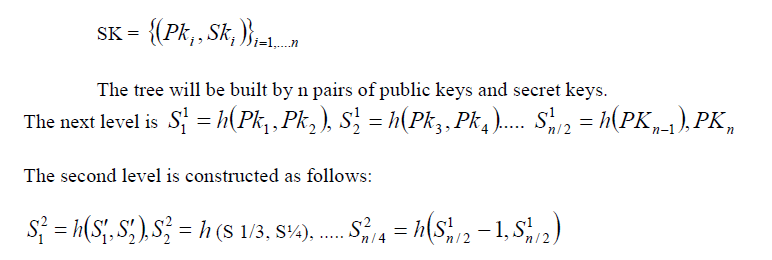A tree is built with one-time signature schemes (PK, SK)s at the nodes. The tree grows from the top to bottom and expensive than the one-time signature scheme. Every time a new pair generated is an expensive task of generating new pairs.

#### 1. Lamport one - Time signature scheme

The security of Lamport signature is based on cryptographic hash function. Here, the public key is used to sign a single message. Other secure hash functions also can be used because this signature scheme is very adjustable. If a hash function becomes insecure, it can be easily exchanged by another secure function. The key generation,signing algorithm and verification algorithm are described as follows.
Key generation
Consider a hash function
H : (0, 1)*
H : (0, 1)s be a cryptographic hash function.
To sign a message M = (0, 1)k and choose 2 * k random numbers Xij with 1 ≤ i ≤ k and j = {0,1}.
For each i and j computeYij= H (Xij).
Here Yijare the public by key and the Yijare the private key values for each 2 * k values.

#### Signing a message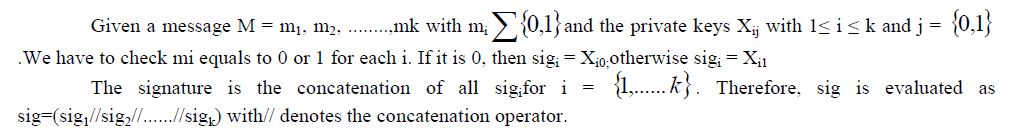#### Signature verification#### 2. Winternitz One-time signature scheme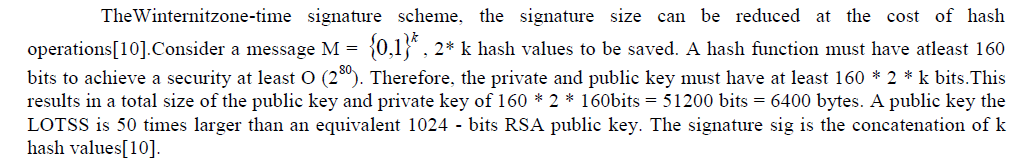#### Key Generation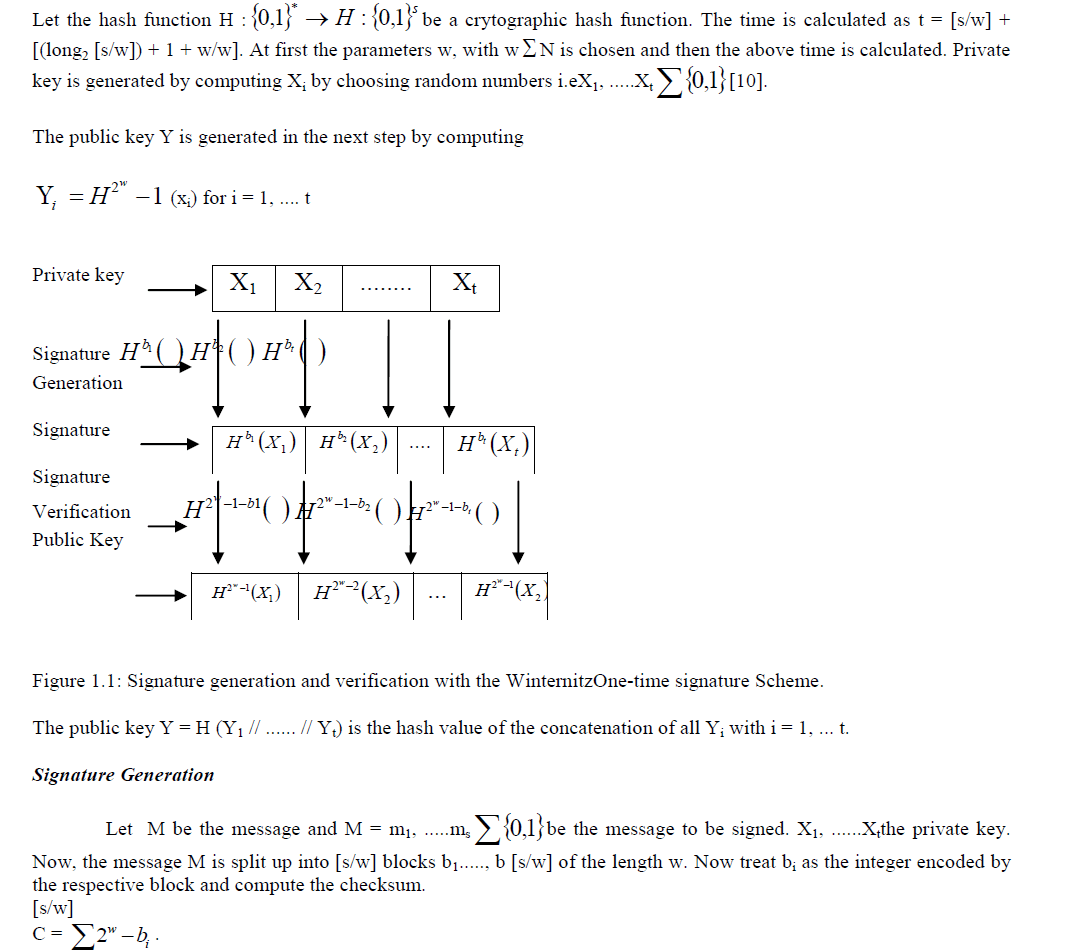We then split the binary representation i = 1of length w. Let us take bi as the integer encoded by the block bi and compute sigi = Hbi (xi) for i = 1,...... t with Ho (xi) = (xi). The signature sig = (sig1//...... // sigt) of the message M is the concatenation of all sigi for i = 1, .....t.

#### Signature verification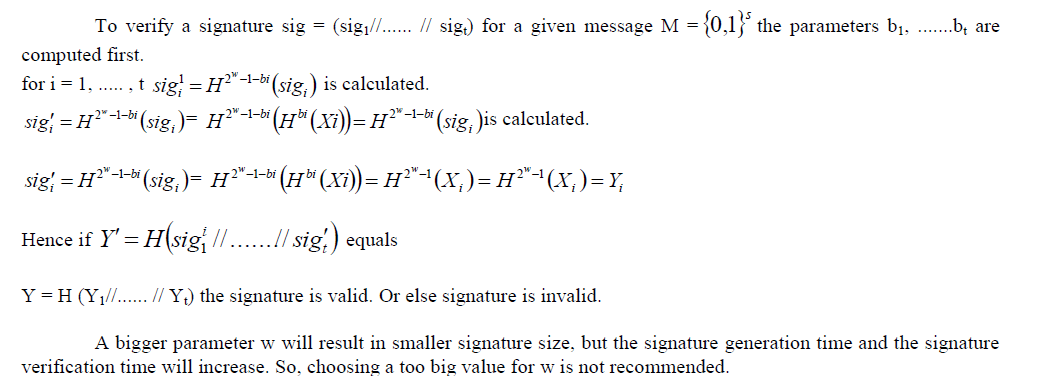### IV. MERKLE - SIGNATURE SCHEME

Key management is the biggest problem in one-time signature scheme. Public key exchanging is very complex and it has not been modified and should be rather short. A new public key is used for every signature and it is quite big in One-time signature scheme. Using an efficient key management, the amount and size of public key used is reduced to make one-time signature scheme feasible. Merkle introduced the Merkle signature Scheme (MSS), in which one public key is used to sign many messages.

#### Key generation

The root of the tree an,o is the public key pub of the Merkle signature scheme. The Merkle Signature Scheme can only be used to sign a limited number of messages with one public key pub. The possible number of messages as N = 2n. The first step of generating the public key pub is to generate the public keys Xi and private keys Yi of 2n one-time signatures for each public key Yi, with 1 ≤ i ≤ 2n, a hash value hi = H (Yi) is computed. With these hash values hi a Merkle tree is build. The node of the tree ai,j, where i denotes the level of the node. The distance between the leaf and node is the level of the node. Therefore, level i=0 is the leaf of the tree and level i=n is the root of the tree.We number all nodes of one level from the left to right, so that ai,ois the left most node of level i. In the Merkle tree, the hash values hi = ai,o. Each inner node of the tree is the hash value of the concatenation of its two children. So,
ai,o= H (ao,o// ao,1) and a2,o = H (a1,o // a1,1).
Therefore, a Merkle tree with 2n leaves and 2n+1- l nodes is build.

#### Signature generation

The Merkle signature scheme, the message M is signed with a one-time signature scheme, resulting in a signature sig1.sig1is evaluated by using one of the public and private key pairs (Xi, Yi). The corresponding leaf of the hash tree to a one-time public key Yi is ao,i= H (Yi). The path of root is A. The path A consists of n+1 nodes i.eAo, ..... An, with Ao = ao,i= pub being the leaf and An = an,0 = pub being root of the tree. To compute this path A, we need every child of the nodes A1, ......An is a child of Ai + 1.The next node is calculated by a brother node called as authi, so that Ai+1 = H (Ai // authi). Hence, to compute every node of the path A, we need brother nodes. These nodes, plus the onetime signature sig' of M is the signature sig = (sig' // auth2 // auth3 // .... authn-1) of the Merkle Signature Scheme.

#### Signature Verification

When the receiver verifies the signature of the message i.esig' ,once if it is valid. Then Ao = H (Yi) is calculated and verified by the receiver. Here, Ao = H (Yi) is hash value of the public key of one-time signature.

### V. CONCLUSION

One-time signature schemes are effective methods, used for key generation and signature verification. The biggest complexity of one-time signature scheme is the key management. The Merkle signature scheme which is used to overcome the problem of reducing the amount and size of the public key and is used to sign many messages. This paper deals detailed study about the importance of one-time signature scheme and Merklesignature scheme on cloud computing.

### REFERENCES

 P. Mell and T. Grance, "Draft NIST working definition of cloud computing".

. Nupoor et al., "Third Party Auditing (TPA) for data storage security in cloud with RC 5 Algorithm", 3(11), pp.1032-1037, 2013.

 Leslie Lamport, "Constructing digital signatures from a one way function". Technical Report SRI-CSL-98, SRI International Computer Science Laboratory, 1979.

 Ralph C. Merkle. A certified digital signature.In Gilles Brassard, editor. CRYPTO, vol 435 of LNCS, pages 218-238, Springer, 1989.

 Chris Dods, Nigel Smart, and MartijnStam, "Hash based digital signature schemes in Cryptography and coding", pages 96-115, Springer, 2005.

 Georg Becker, "Merkle signature schemes, Merkle Trees and their Cryptanalysis", pp. 1-24, 2008.

 Heiser J. (2009) what you need to know about cloud computing security and compliance,Gartner, Research, ID Number : G00168345.

 Amzon Elastic compute cloud (ECZ). <http://aws.amazon.com/secuirty Accessed: [January 2013].

 Choudary V (2007). Software as a service: implications for investment in software development in: International conference on system sciences, pp. 209,2007.

“https://www.emsec.rub.de/media/crypto/attachments/files/2011/04/becker_1.pdf”.